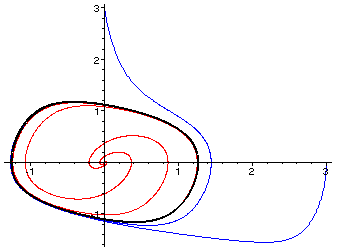Templates for ( Math 4 )

Here is some help regarding down loading maple 7 to a Mac and here is some help for loading it onto a PC .

Our first template can be used to solve a first order differential equation with one dependent and one independent variable. In this case we are solving

dy/dt=-y^2+5sin(t)
subject to the initial data that at time .2 y's value is 5.

Here is an example of using this template to explore the standard exponential function ant it relation to dY/dt=Y giving us a nice use of differential equations to approximate the constant e.

Here is an example were we explore some possible parameter changes when modeling fish populations.

Our second template can be used to solve a first order system of differential equations.

Here is our initial use of the logistic equation in order to model a fish population. Here we make the model more accurate wit ha periodic periodic birth rate. Here is model we develop for a fish population that was experiencing the effects of pollution (as well as a seasonally determined birth rate).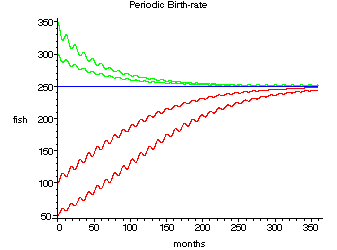Here is the Lokta-Volterra type model of our fish and fisher folk and here is the alligator model. Here are the three models for a "ecosystem" involving fish, alligators and fisher-folk that you developed in class JPAK , STA ,and AMRN .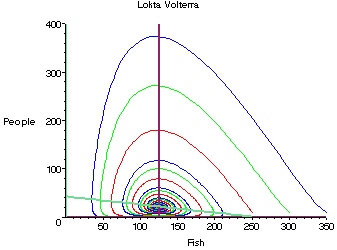Here is how to make a legend. in the context of our Lokta-Volterra model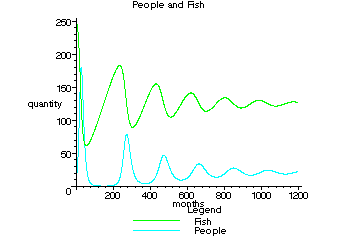At this point we are making a detailed exploration of the equation
dx/dt=x(1-x)-axy

dy/dt=-y+axy

Part of or motivation is as we vary the parameter a from 1.1 to 2 we see a bifurcation , in other words a qualitative change in the systems behavior. In order to understand this we zoom into the equilibrium and notice that near its equilibrium point the solution to this equation really does behave like those of the equation's linearization . Fortunately (up to to a wiggle) we can determine the qualitative properties of the solution from its linearization, and in this program we see how to take a system along with a known equilibrium point and find its linearization and explore this linear systems eigenvalues. Recall the linear system's eigenvalues allow us to determine the original system's qualitative properties near the equilibrium point by comparing it to a standard linear model.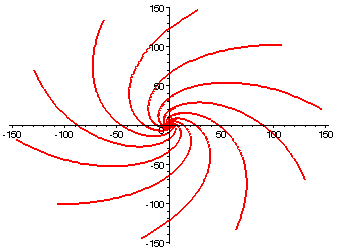Here is a simulation of the final epidemic model form the De Leo and Dobson paper. Here are the simulations of the variations to the model that we discussed in lecture article , variation 1 , variation 2 , and variation 3 (for a couple of different parameter choices). Here is a simulation for the fast moving non-lethal epidemics as discussed in class.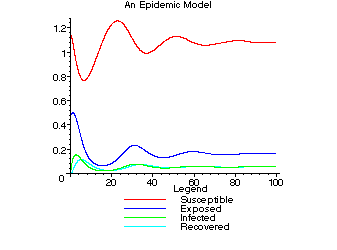Here we explore the damped oscillator and here see what happen as we over damp the system with as we vary the friction . Here we explore the van der Pol equation. Here see the meaning of a relaxation oscillation as we vary an interesting parameter in the van der Pol equation.